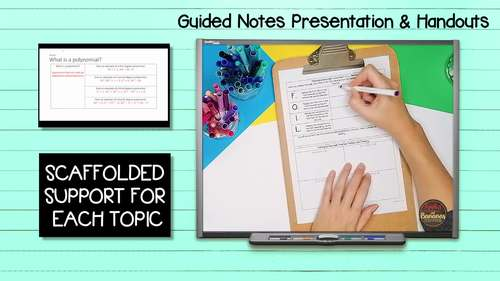# Introduction to Polynomials - Guided Notes, Presentation, and INB Activities

Rated 5 out of 5, based on 33 reviews
33 Ratings;
7th - 10th, Homeschool
Subjects
Resource Type
Standards
Formats Included
• Zip
Pages
37 pages
Report this resource to TPT
##### Also included in
1. This Algebra 1 Bundle is flexible, editable, and can be used for in-person or distance learning. Take the time and stress out of creating your own guided notes and presentations. There are many ways to use this engaging bundle. Students can either build interactive math notebooks with guided notes (
Price \$55.60Original Price \$69.50Save \$13.90
2. This HUGE (over 1,000 pages) high school bundle contains a full-year of guided/scaffolded notes and interactive notebook activities for an entire year of Algebra 1, Geometry, and Algebra 2. The Algebra 1 bundle contains 11 units of study plus 2 mini-units, the Geometry bundle contains 13 units, and
Price \$180.74Original Price \$258.20Save \$77.46
3. This HUGE (over 500 pages) Algebra curriculum pack contains three of our full-year, best selling bundles: Guided Notes Bundle (scaffolded notes, presentation/presentation handouts, INB activities, quick checks for understanding), Student Practice Pages Bundle, and Algebra 1 Assessments Bundle. All
Price \$90.72Original Price \$129.60Save \$38.88
4. This product contains three full years of editable content (Algebra 1, Geometry, Algebra 2) for the high school mathematics classroom. It includes Guided Notes Bundles (guided notes, presentation, presentation handouts, INB foldable activities, and quick checks for understanding), Student Practice P
Price \$320.15Original Price \$492.55Save \$172.40

### Description

This flexible resource on Introduction to Polynomials allows students to either build interactive math notebooks with guided notes (keys included) and foldable activities OR use the included presentation handouts (keys included) with the PowerPoint presentation for focused instruction. Quick checks for understanding (keys included) help to determine how well your students understand the material as you go. Choose what works best for your class and modify to make the content fit your needs. Notes are designed to clearly present the topics and reach all types of learners.

The zip file includes BOTH editable, plain-font resources and ready-to-print, non-editable PDFs. Suggested use guides are included.

Interactive Math Notebook FORMAT – Includes 3 parts: guided notes, foldable activities, and quick checks for understanding. Piece the components together and students can create their own “math textbook” in a math journal.

Presentation Sheets FORMAT – Include 2 parts: guided notes and quick checks for understanding. They are organized in the same order as the included PowerPoint and are designed to print and present. The quick checks can be cut off the bottom of the note sheets and turned in as an assessment tool.

Presentation – Plain-font, no frills, PowerPoint presentation that you can use to present content to students. Slides have blank, student note templates followed by filled in teacher keys. Modify the editable presentation to best fit your needs.

• What is a Polynomial?
• How Do You Add Two Polynomial Expressions?
• How Do You Subtract Two Polynomial Expressions?
• Polynomial Equations with Addition and Subtraction
• Distributive Property
• Polynomial Equations with the Distributive Property
• What is factoring?
• Multiplying Binomials Using the F.O.I.L Method
• Multiplying Binomials Practice: Multiply and Simplify
• Factoring Trinomials
• Factoring Trinomials with the “X” Method
• Extra Tips for Factoring Trinomials
• More Factoring: The Difference of Two Square Terms
• What if You Have to Find the Product of a Monomial and Two Binomials?
• Solving Quadratic Equations with Factoring

Introduction to Polynomials Student Practice Pages: One full page of practice for each topic covered in the quick checks for understanding. Includes student pages and teacher keys. Perfect for homework, classwork, or a review.

Introduction to Polynomials Editable Assessments: Two editable assessments that pair perfectly with the unit.

Related Products:

**Full-year Curriculum Packs**

Algebra 1, Geometry, Algebra 2 Curriculum Pack MEGA BUNDLE

Algebra 1 Curriculum Pack BUNDLE

Geometry Curriculum Pack BUNDLE

Algebra 2 Curriculum Pack BUNDLE

Scaffolded Notes/Interactive Notebook Bundles

Algebra 1 INB Bundle

Geometry INB Bundle

Algebra 2 INB Bundle

Pre-Calculus INB Bundle

Pre-Calculus Add on for Algebra 2 INB Bundle

Statistics INB Bundle

High School Math Interactive Notebook Bundle (Algebra 1, Geometry, Algebra 2)

Student Practice Pages Bundles

Algebra 1 Student Practice Pages Bundle

Geometry Student Practice Pages Bundle

Algebra 2 Student Practice Pages Bundle

High School Math Student Practice Pages Bundle (Algebra 1, Geometry, Algebra 2)

Assessment Bundles

Algebra 1 Assessment Bundle

Geometry Assessment Bundle

Algebra 2 Assessment Bundle

High School Math Assessment Bundle (Algebra 1, Geometry, Algebra 2)

Consumer Math

Consumer Math Bundle

**If you like what you see, please click on the "Follow Me" star to learn about new products, sales, and more!

Total Pages
37 pages
N/A
Teaching Duration
N/A
Report this resource to TPT
Reported resources will be reviewed by our team. Report this resource to let us know if this resource violates TPT’s content guidelines.

### Standards

to see state-specific standards (only available in the US).
Understand that polynomials form a system analogous to the integers, namely, they are closed under the operations of addition, subtraction, and multiplication; add, subtract, and multiply polynomials.
Identify zeros of polynomials when suitable factorizations are available, and use the zeros to construct a rough graph of the function defined by the polynomial.
Prove polynomial identities and use them to describe numerical relationships. For example, the polynomial identity (𝘹² + 𝘺²)² = (𝘹² – 𝘺²)² + (2𝘹𝘺)² can be used to generate Pythagorean triples.
Understand that rational expressions form a system analogous to the rational numbers, closed under addition, subtraction, multiplication, and division by a nonzero rational expression; add, subtract, multiply, and divide rational expressions.
Use the process of factoring and completing the square in a quadratic function to show zeros, extreme values, and symmetry of the graph, and interpret these in terms of a context.